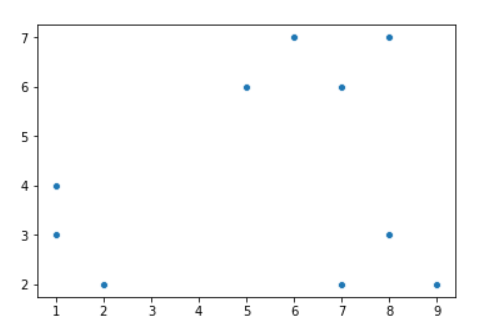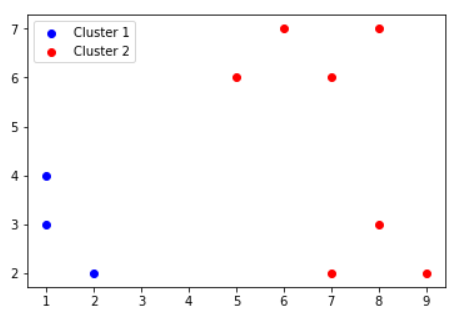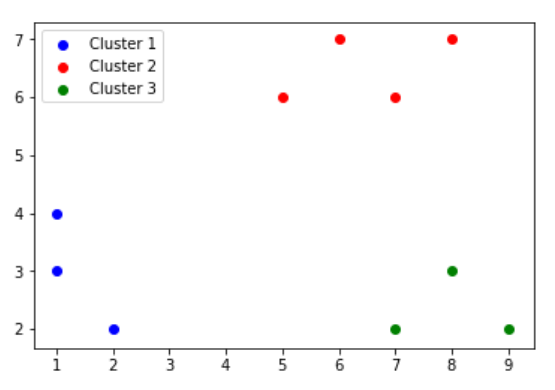×

Search anything:

# K+ Means Clustering algorithm

#### clustering algorithm data mining Machine Learning (ML) k means k+ meansIn case you haven't read about the K Means Algorithm yet, it is highly recommended to first go through it, in order to understand best, how K+ means works.

K-means is one of the most popular and simplest unsupervised learning algorithms
that solve the well-known clustering problem. The procedure follows a simple and easy way to classify a given data set to a predefined, say K number of clusters.

However, a major drawback of K Means is, that we need to determine the optimal values of K, and this is a very difficult job. Prior to solving the problem, it is difficult to know which value of K would do best for our particular problem.

To overcome this difficult problem, we use the K+ Means algorithm, which incorporates a method to calculate a good value of K for our problems. K+ Means algorithm builds onto K Means algorithm, and is an enhancement to it.

K+ Means is also great at detecting outliers, and forming new clusters for them. Therefore, K+ Means is not sensitive to outliers, unlike the K Means algorithm.

### The K+ Means algorithm

STEP 1: Find K clusters using the K-Means algorithm
STEP 2: For each of the clusters, calculate MIN, AVG and MAX intra cluster dissimilarity
STEP 3: If, for any cluster, AVG value is greater than others, then MIN and MAX values are checked. If the MAX value is higher too, we assign the corresponding data point as a new cluster and go back to step 1, with K+1 clusters.
STEP 4: Repeat STEPS 1 to 3 until no new clusters are formed.

### What is intra-cluster dissimilarity?

Intra-cluster dissimilarity, or intra-cluster distance, is a metric to find the distances of each data point from the centroid of the cluster, in order to detect outliers.

MIN: Related to point closest to centroid
MAX: Related to point furthest away from centroid (potential outlier)
AVG: Average of distances of all points with centroid respectively.

All distances will be calculated as Euclidean distances

## Example

Let's assume 10 input data points, with points labeled from P1 to P10. We will apply our K+ means algorithm on this input data, in order to understand the algorithm better:

Point X Y
P1 1 4
P2 1 3
P3 2 2
P4 7 2
P5 8 3
P6 9 2
P7 5 6
P8 6 7
P9 7 6
P10 8 7

Here's how a scatter plot of our input data looks:We will assume K=2 for this example.
Our initial centroids will be P1 and P5

STEP 1: Find K clusters using the K means algorithm.

We will initially run K-means algorithm, to get the following as the 2 clusters:

Clusters Points
Cluster1 {P1,P2,P3}
Cluster2 {P4,P5,P6,P7,P8,P9,P10}STEP 2: For each of the clusters, calculate MIN, AVG and MAX intra cluster dissimilarity.

Cluster 1:
For finding intra-cluster distances, we first will need to find the centroid G1.
G1 = ( 1.33 , 3 )

Point X Y Distance from G1
P1 1 4 1.05
P2 1 3 0.33
P3 2 2 1.20

Hence,
MIN = 0.33
MAX = 1.20
AVG = 0.86

Cluster 2:
For finding intra-cluster distances, we first will need to find the centroid G2.
G2 = ( 7.14 , 4.71 )

Point X Y Distance from G1
P4 7 2 2.71
P5 8 3 1.91
P6 9 2 3.29
P7 5 6 2.50
P8 6 7 2.56
P9 7 6 1.30
P10 8 7 2.45

Hence,
MIN = 1.30
MAX = 3.29
AVG = 2.39

STEP 3: If, for any cluster, AVG value is greater than others, then MIN and MAX values are checked. If the MAX value is higher too, we assign the corresponding data point as a new cluster and go back to step 1, with K+1 clusters.

Here, we can clearly see that AVG value of Cluster 2 is much greater than Cluster 1, so we'll compare the MAX values now:

Since 3.29 > 1.20 by a significant margin, we can confirm that Cluster 2 has the presence of an outlier.

MAX value of 3.29 is present in the P6 data point, so that will be the point we can remove from Cluster 2.

STEP 4: Repeat STEPS 1 to 3 until no new clusters are formed.

Clusters Points
Cluster1 {P1,P2,P3}
Cluster2 {P4,P5,P7,P8,P9,P10}
Cluster2 {P6}Now, we perform STEP 1 again, to form the following clusters:

Clusters Points
Cluster1 {P1,P2,P3}
Cluster2 {P7,P8,P9,P10}
Cluster2 {P6,P4,P5}

Again, we perform STEP 2:

Cluster 1 (unchanged):
MIN = 0.33
MAX = 1.20
AVG = 0.86

Cluster 2:

New G2 = (6.5 , 6.5)

Point X Y Distance from G2
P7 5 6 1.58
P8 6 7 0.71
P9 7 6 0.71
P10 8 7 1.58

MIN = 0.71
MAX = 1.58
AVG = 1.145

Cluster 3:

G3 = (8,2.33)

Point X Y Distance from G1
P4 7 2 1.05
P5 8 3 0.67
P6 9 2 1.05

MIN = 0.67
MAX = 1.05
AVG = 0.92

STEP 3:
Cluster 1,2 and 3, we find that the AVG inter-cluster distances are quite similar. Hence, we can safely say that there are no outliers, and that these are our final 3 clusters.

FINAL CLUSTERS:

Clusters Points
Cluster1 {P1,P2,P3}
Cluster2 {P7,P8,P9,P10}
Cluster2 {P6,P4,P5}### Complexity analysis

Complexity of K Mean algorithm = O(tkn), where:

• t is the number of iterations
• k is the number of clusters
• n is the number of data points

K+ Mean algorithm is computationally more expensive as compared to K Means, since it performs a few extra steps in order to find the better value of K.

However, the simplicity and effectiveness of K+ Means more than makes up for the time complexity.

Complexity of K+ Mean algorithm = O(t * (k^2) * n).

## Conclusion

The K+ Means algorithm takes all the strengths of the widely popular K Means algorithm and remains simple and easy to use and understand. In addition, it removes the weaknesses of the K-Means algorithm as well.

## Reference paper

K+ Means : An Enhancement Over K-Means Clustering Algorithm